The HPMIXED Procedure
 OUTPUT Statement
OUTPUT <OUT=SAS-data-set>
<keyword<(keyword-options)> <=name>>...
<keyword<(keyword-options)> <=name>> </ options>
;
The OUTPUT statement creates a data set that contains predicted values and residual diagnostics, computed after fitting the model. By default, all variables in the original data set are included in the output data set.

You can use the ID statement to select a subset of the variables from the input data set to be added to the output data set.

For example, suppose that the data set Scores contains the variables score, machine, and person. The following statements fit a model with fixed machine and random person effects and save the predicted and residual values to the data set igausout:

```proc hpmixed data = Scores;
class machine person score;
model score = machine;
random person;
output out=igausout pred=p resid=r;
run;
```
You can specify the following options in the OUTPUT statement before the slash (/).
OUT=SAS data set
DATA=SAS data set

specifies the name of the output data set. If the OUT= (or DATA=) option is omitted, the procedure uses the DATAn convention to name the output data set.

keyword <(keyword-options)><=name>

specifies a statistic to include in the output data set and optionally assigns the variable the name name. You can use the keyword-options to control which type of a particular statistic to compute. The keyword-options can take on the following values:

BLUP

uses the predictors of the random effects in computing the statistic.

NOBLUP

does not use the predictors of the random effects in computing the statistic.

The default is to compute statistics by using BLUPs. For example, the following two OUTPUT statements are equivalent:

```output out=out1  pred=predicted lcl=lower;
output out=out1  pred(blup)=predicted lcl(blup)=lower;
```

If a particular combination of keyword and keyword options is not supported, the statistic is not computed and a message is produced in the SAS log.

A keyword can appear multiple times in the OUTPUT statement. Table 43.4 lists the keywords and the default names assigned by the HPMIXED procedure if you do not specify a name. In this table,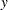denotes the response variable.

Table 43.4 Keywords for Output Statistics

Keyword

Options

Description

Expression

Name

PREDICTED

BLUP

Linear predictor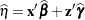Pred

NOBLUP

Marginal linear predictor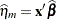PredPA

STDERR

BLUP

Standard deviation of linear predictor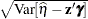StdErr

NOBLUP

Standard deviation of marginal linear predictor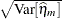StdErrPA

RESIDUAL

BLUP

Residual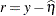Resid

NOBLUP

Marginal residual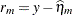ResidPA

PEARSON

BLUP

Pearson-type residual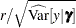Pearson

NOBLUP

Marginal Pearson-type residual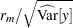PearsonPA

STUDENT

BLUP

Studentized residual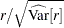Student

NOBLUP

Studentized marginal residual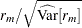StudentPA

LCL

BLUP

Lower prediction limit for linear predictor

LCL

NOBLUP

Lower confidence limit for marginal linear predictor

LCLPA

UCL

BLUP

Upper prediction limit for linear predictor

UCL

NOBLUP

Upper confidence limit for marginal linear predictor

UCLPA

VARIANCE

BLUP

Conditional variance of response variable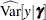Variance

NOBLUP

Marginal variance of response variable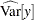VariancePA

You can use the following shortcuts to request statistics: PRED for PREDICTED, STD for STDERR, RESID for RESIDUAL, VAR for VARIANCE.

You can specify the following options of the OUTPUT statement after the slash (/).

ALLSTATS

requests that all statistics are computed. If you do not use a keyword to assign a name, the HPMIXED procedure uses the default name.

ALPHA=number

determines the coverage probability for two-sided confidence and prediction intervals. The coverage probability is computed as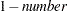. The value of number must be between 0 and 1 inclusively; the default is 0.05.

NOMISS

requests that records from the input data set be written to the output data only for those observations that were used in the analysis. By default, the HPMIXED procedure produces output statistics for all observations in the input data set.

NOUNIQUE

requests that names not be made unique in the case of naming conflicts. By default, the HPMIXED procedure avoids naming conflicts by assigning a unique name to each output variable. If you specify the NOUNIQUE option, variables with conflicting names are not renamed. In that case, the first variable added to the output data set takes precedence.

NOVAR

requests that variables from the input data set not be added to the output data set. This option ignores ID statement but does not apply to variables listed in a BY statement.Previous Page | Next Page | Top of Page